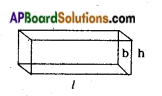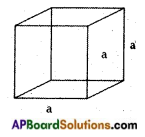# AP Board 8th Class Maths Notes Chapter 14 Surface Areas and Volume

Students can go through AP Board 8th Class Maths Notes Chapter 14 Surface Areas and Volume to understand and remember the concepts easily.

## AP State Board Syllabus 8th Class Maths Notes Chapter 14 Surface Areas and Volume

→ If l, b, h are-the dimensions of cuboid, then:(i) its lateral surface area is 2h (l+ b)
(ii) its total surface area is 2 (lb + bh + hl)
(iii) its volume is l × b × h

→ Lateral surface area of a cube is 4a2→ Total surface area of a cube is 6a2→ Volume of a cube is side × side × side = a3

→ 1 cm3 = 1 ml

→ 1 l = 1000 cm3

→ 1 m3 = 1000000 cm3 = 1000 l = 1 kl (kilolitre)